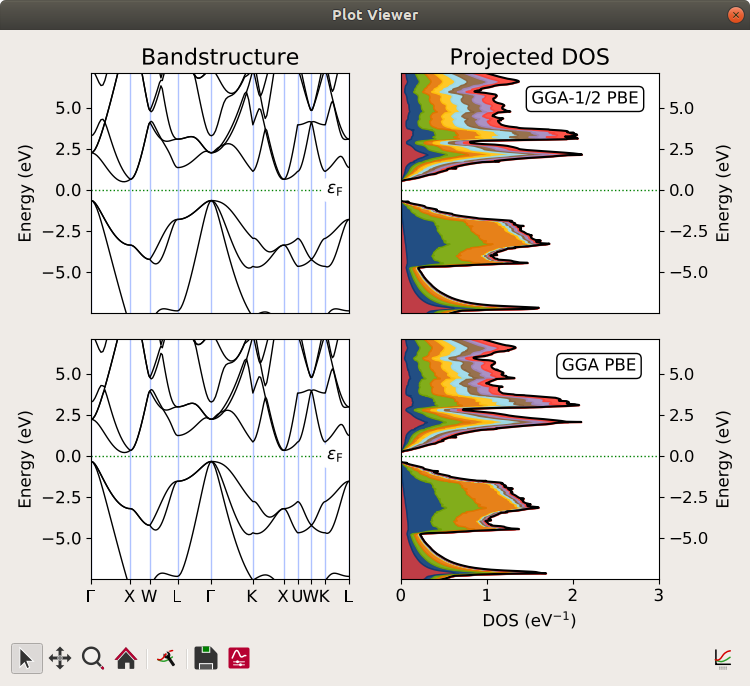# Editing, Saving and Merging Plots¶

Version: Q-2019.12

This guide shows you how to take advantage of NanoLab plotting capabilities to customize, save, merge and re-use plots.

The QuantumATK NanoLab plugins allow you to produce a large variety plots that visualize Bandstructures, Projected Density of States (PDOS), ElectronPhononCouplings, Potentials, etc. Each of these plots can be edited, saved and loaded witin NanoLab. Plots can also be merged together in compound plots that visualize complex relationships between configurations, calculations and analyses. Lastly, those compound plots can be re-used for other calculations and results.

Note

As a simple example this guide uses the Projected DOS and Bandstructure analyses calculated for bulk silicon with a LCAO calculator using the GGA-1/2 PBE exchange correlation.

## Video guide¶

This is a video guide showcasing the NanoLab plotting capabilities. Detailed information is provided in the written guide below.

## Plot tools¶

To facilitate easy editing, all plots are accompanied by a plot tool bar. The toolbar contains these tools:

1.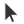Interaction tool, where items can be selected. Right-click on a plot to add arrows, measures and labels to the plot.
2.Pan tool. Left-click and drag a plot to change the axis limits. Right-click and drag to zoom.
3.Zoom tool. Right-click and drag to draw a zoom-rectangle. Use the mouse wheel to zoom in and out.
4.Editor tool. Click this tool bring up the Plot Editor.
5.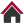Home tool. Clicking this tool resets the current axes limits.
6.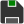Save tool. Click to save the interactive plot in ‘hdf5’, export data to ‘txt’, or export the plot as an image in e.g. ‘pdf’ or ‘png’ formats.
7.Merge tool. Drag this icon to merge the plot with itself or other plots.

The PDOS (Projected Density of States) Analyzer plots the density of states projected onto elements, orbitals or shells. For our example bulk silicon calculation the PDOS analyzer looks like this: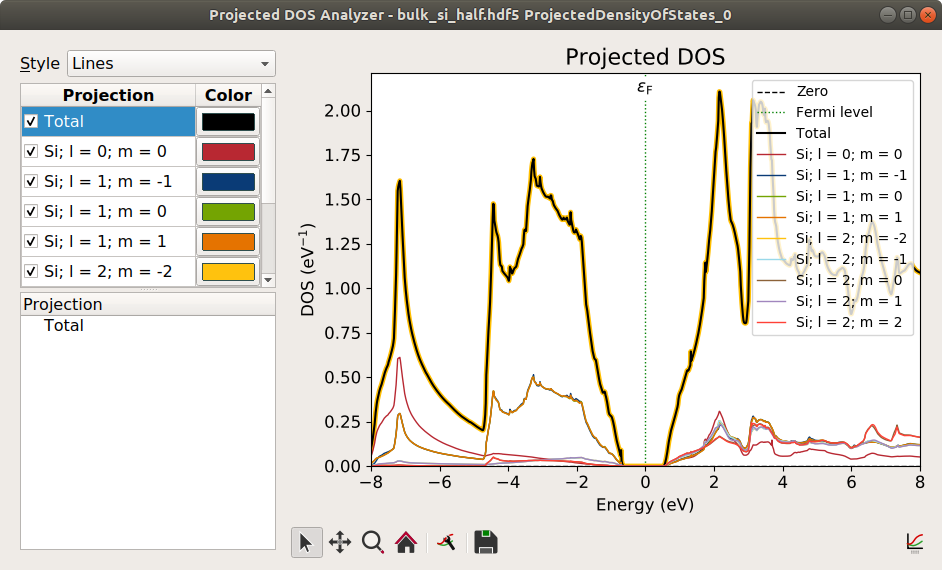##Annotate plots¶

The Interaction tool shows helpful tool tips when hovering over different plot items. Once hovered, left clicking selects the item. Right clicking brings up the annotation menu with these options:

When right clicking on a line, the menu also includes these additional options:

Tip

For our example Project DOS plot, one common analysis is to measure the band gap. This can be done by inserting avertical measure into the plot. The meausure can then be “snapped” to the lines near the band edges.

##The Plot Editor¶

Launch the plot editor from the toolbar or by pressing “E”. The plot editor makes it possible to edit the individual properties of the layout, legend, title, axes, and lines of the plot.

The editor lists all the plot components. At the top level all plots are built from aGridLayout. The layout then contains one (or multiple)Plot Frames. The dimensions of the grid and the position of the individual frames can all be adjusted in the Plot Editor.

Each frame has atitle, alegend, anx-axis and ay-axis, and may also contain data from one or moresources. Simply select the different components to edit their color, line-width, text, opacity and so forth.

One such source could be our Projected DOS plot, which consists of some projection lines, a vertical Fermi line and a Fermi label. You can select multiple lines and edit all their properties at once.The editor also has a few additional tools:

1.Zoom to item. Select a single item and zoom to that item.
2.Add analysis. Lines support additional analysis that you may add here. You can choose between polynomial fits, gaussian fits, or specify your own fitting function in the curve fit. You can also add an average or a rolling average analysis.
3.Export data. Select the relevant plot items and choose the “save” icon. This will export data to txt format.

##Save and load the plot in the PlotViewer¶

After editing the plot, you can save it to a file in the ‘hdf5’ format. In the file browser MainWindow you can then “check” the newly created file and the Plot will appear on the Lab floor. Open the Plot using the PlotViewer plugin in the Analysis Bar located to the right of the Lab floor.

The opened plot contains all your exiting modifications. You can continue editing, or you canre-open the original analyzer (in our example this would be the PDOS analyzer).

To re-open the analyzer, click the red analyzer icon. This tries to open the original PDOS analyzer. Sometimes the original analysis object is required in order to re-open the analyzer. If the analysis object is missing you can replace the data by dragging and dropping it from the Lab floor. See also the section about “Redo analysis”.

Note

In our case, we may wish to change from lines to filled stacked lines. This could be done in the PDOS analyzer, so we re-open the analyzer and choose the “Stacked” style. The PDOS plot is then immediately updated.

##Merge plots¶

All plots can be merged together by dragging and dropping the plots onto each other. In a plot you can “grab” the plot by grabbing themerge icon in the plot toolbar, and on the Lab floor you simply grab the Lab floor icon.

In general the plotting framework supports two different ways of merging plots together depending on where you drop the plot.

### Drop next to, above or below an existing plot¶

When dragging a plot, you can drop it on four “green” areas at the edges of a plot. This will create a new plot containing the original plot along with the dropped plot. Once merged both plots can still be edited and adjusted and their analyzers can still be re-opened.

In our example we also calculated the Projected DOS for bulk silicon with DFT-1/2 turned off. Dropping this PDOS plot next to the GGA-1/2 PDOS plot clearly shows that the two calculations produce a different band gap. Add two horizontal measures and adjust the titles to produce the plot below: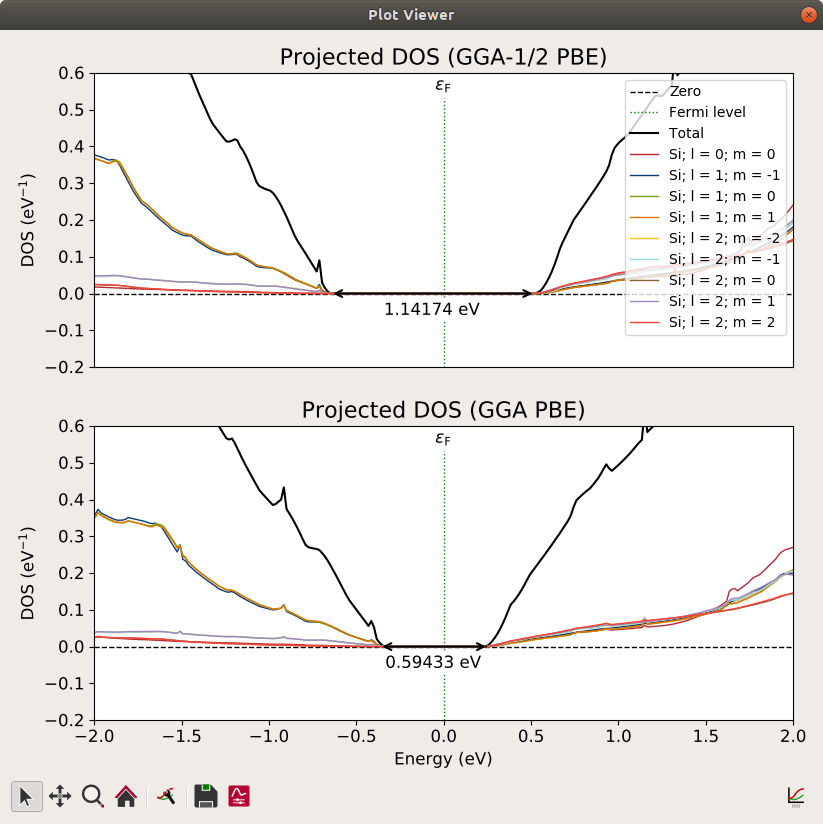Note

Note that the DFT-1/2 result is very close to the experimentally measured band gap of bulk silicon of 1.14 eV.

### Drop on the existing plot¶

You can also drop a plot on the middle of another/the same plot. This offers a dialog with some advanced merge options. Depending on the units on the axes of your merged plots, you may have the following options:

1. Overlay with one or two shared axes.
2. Flip the plot and then overlay.
3. “Mirror” (plot next to each other but with linked axes)
4. Flip the plot and then mirror.

For bulk silicon we also calculated the Bandstructure, and we want to inspect the calculated Projected DOS and Bandstructure next to each other. We grab the“merge” icon in the Bandstructure plot and drop it on the PDOS plot. In the dialog we choose the option “Flip and mirror with shared y-axis”.

This creates the following merged plot where the y-axes (the energy axes) on both plots have been linked.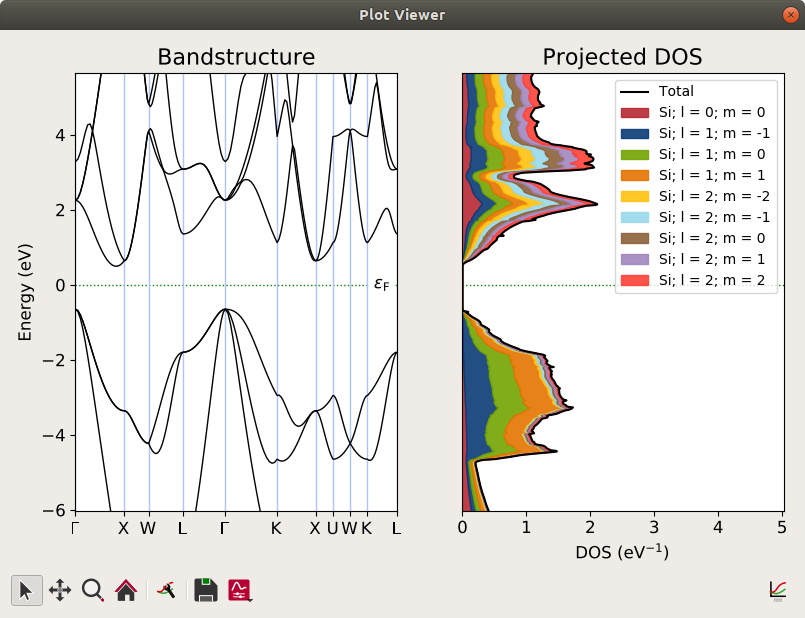##Redo analysis¶

By merging plots from multiple QuantumATK NanoLab plugins it is possible to create interesting compound plots showing different aspects of your calculated data. In our example the merged plot of the Bandstructure and Projected DOS is one such a plot.

NanoLab allows you to reuse a compound plot. This means that you may easily redo this analysis for another set of calculated results. It is possible to replace data in the following way:

1. Select the data on the Lab floor.
2. Drop the data in the middle of the plot.
3. The plots are then updated with the new data.

You can also create two plots side by side for an easy comparison.

1. Select the data on the Lab floor.
2. Drop the data on the edge of the plot.
3. A new plot is created with the original and the new plot side by side.

In our analysis of bulk silicon we would e.g. select the PDOS and Bandstructure Lab floor items from the GGA PBE calculation (DFT-1/2 disabled) and drop them next to our merged plot. In the compound plot we edited the titles and added two labels to produce the following comparison.